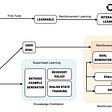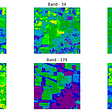# All machine learning models explained

As a beginner, you want to know what are the models and algorithms available in machine learning that make our work more easier.

so in this article, we would be knowing about all of the machine learning model used which a beginner should know.

as machine learning models majorly fall into 2 subclasses, and then supervised learning is further subcategorized as either a regression or classification model.

# supervised learning

Supervised learning involves learning a function that maps an input to an output based on example input-output pairs.

# Regression

In regression models, the output is continuous.

## Linear regression

The idea of linear regression is simply finding a line that best fits the data. Extensions of linear regression include multiple linear regression.

## Decision tree

Decision trees are a popular model, used in operations research, strategic planning, and machine learning. Each circle below is called a node, and the more nodes you have, the more accurate your decision tree will be(generally). The last nodes of the decision tree, where a decision is made, are called the leaves of the tree

## Random forests

Random forests are an ensemble learning technique that builds off of decision trees. Random forests involve creating multiple decision trees using bootstrapped datasets of the original data and randomly selecting a subset of variables at each step of the decision tree. The model then selects the mode of all of the predictions of each decision tree

## neural networks

A Neural Network is essentially a network of mathematical equations. It takes one or more input variables, and going through a network of equations, results in one or more output variables.

# classification

In classification models, the output is discrete.

## logistic regression

Logistic regression is similar to linear regression but is used to model the probability of a finite number of outcomes, typically two.

## support vector machine

A support vector machine will find a hyperplane or a boundary between the two classes of data that maximizes the margin between the two classes. Many planes can separate the two classes, but only one plane can maximize the margin or distance between the classes.

## Naive Bayes

Naive Bayes is another popular classifier used in Data Science The idea behind it is driven by Bayes Theorem .the goal is to find the classy with the maximum proportional probability.

## Decision Tree, Random Forest, Neural Network

the same as above

# unsupervised learning

Unlike supervised learning, unsupervised learning is used to draw inferences and find patterns from input data without references to labeled outcomes.

## clustering

Clustering is an unsupervised technique that involves the grouping, or clustering, of data points. It’s frequently used for customer segmentation, fraud detection, and document classification. Common clustering techniques include k-means clustering, hierarchical clustering, mean shift clustering, and density-based clustering.

## principal component analysis

Principal Component Analysis (PCA) is used to make data easy to explore and visualize by reducing the number of variables. This is done by capturing the maximum variance in the data into a new coordinate system with axes called principal components.

## conclusion

in this article I hope, you guys have a basic knowledge of all the common machine learning models used in the future articles I would be telling you more about each and every model and doing a hands-on project on them.

--

--

--

## More from Aviral Bhardwaj

writer

Love podcasts or audiobooks? Learn on the go with our new app.

## Efficiently reading large volumes of data from redshift with pyarrow## Conversational AI — Key Technologies and Challenges — Part 2## Bob Hayes - A terrific blogger, fitness freak, Data Science expert## Most charities want to get more from their data## CD Studio: visualizing patterns## Do EastEnders fans like Hollyoaks? Analysis in R## Hyperspectral Image Analysis — Classificationwriter

## Building an Expected Goals Soccer Model using Machine Learning Decision Trees and XGBoost## Understanding classification and clustering in machine learning## Cervical Cancer Prediction Using Machine Learning## Introduction to Machine Learning